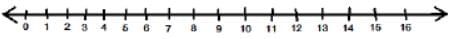Test: Addition and Subtraction Up to 20- 2

# Test: Addition and Subtraction Up to 20- 2

Test Description

## 10 Questions MCQ Test Mathematics for Class 1 | Test: Addition and Subtraction Up to 20- 2

Test: Addition and Subtraction Up to 20- 2 for Class 1 2023 is part of Mathematics for Class 1 preparation. The Test: Addition and Subtraction Up to 20- 2 questions and answers have been prepared according to the Class 1 exam syllabus.The Test: Addition and Subtraction Up to 20- 2 MCQs are made for Class 1 2023 Exam. Find important definitions, questions, notes, meanings, examples, exercises, MCQs and online tests for Test: Addition and Subtraction Up to 20- 2 below.
Solutions of Test: Addition and Subtraction Up to 20- 2 questions in English are available as part of our Mathematics for Class 1 for Class 1 & Test: Addition and Subtraction Up to 20- 2 solutions in Hindi for Mathematics for Class 1 course. Download more important topics, notes, lectures and mock test series for Class 1 Exam by signing up for free. Attempt Test: Addition and Subtraction Up to 20- 2 | 10 questions in 15 minutes | Mock test for Class 1 preparation | Free important questions MCQ to study Mathematics for Class 1 for Class 1 Exam | Download free PDF with solutions
 1 Crore+ students have signed up on EduRev. Have you?
Test: Addition and Subtraction Up to 20- 2 - Question 1

### The sum of 4 + 5 is

Detailed Solution for Test: Addition and Subtraction Up to 20- 2 - Question 1

4 + 5 = 9

Test: Addition and Subtraction Up to 20- 2 - Question 2

### Which rectangle matches the number in the circle? (7)

Detailed Solution for Test: Addition and Subtraction Up to 20- 2 - Question 2

(a) 3 + 2 = 5
(b) 2 + 2 = 4
(c) 3 + 4 = 7
(d) 8 + 0 = 8

Test: Addition and Subtraction Up to 20- 2 - Question 3

### I am a number just after 3 + 0

Detailed Solution for Test: Addition and Subtraction Up to 20- 2 - Question 3

3 + 0 = 3
Therefore, number just after 3 is 4

Test: Addition and Subtraction Up to 20- 2 - Question 4

Use the number line to add 9 + 5.Detailed Solution for Test: Addition and Subtraction Up to 20- 2 - Question 4

9 + 5 = 14

Test: Addition and Subtraction Up to 20- 2 - Question 5

Tick the correct one

Detailed Solution for Test: Addition and Subtraction Up to 20- 2 - Question 5

(a) 3 + 0 = 3
(b) 4 + 4 = 8
(c) 5 + 5 = 12
(d) 3 + 3 = 7

Test: Addition and Subtraction Up to 20- 2 - Question 6

10 girls are jumping rope. 10 more girls join them. Now, how many girls are jumping rope

Detailed Solution for Test: Addition and Subtraction Up to 20- 2 - Question 6

10 + 10 = 20

Test: Addition and Subtraction Up to 20- 2 - Question 7

The sum of 2 + 3 + 2 is

Detailed Solution for Test: Addition and Subtraction Up to 20- 2 - Question 7

2 + 3 + 2 = 8

Test: Addition and Subtraction Up to 20- 2 - Question 8

Compare. 15 + 0 ( )17 + 1

Detailed Solution for Test: Addition and Subtraction Up to 20- 2 - Question 8

15 + 0 = 15
17 + 1 = 18
Therefore, 15 + 0 < 17 + 1

Test: Addition and Subtraction Up to 20- 2 - Question 9

What number is 5 more than 50?

Detailed Solution for Test: Addition and Subtraction Up to 20- 2 - Question 9

We are looking for a new number which is 5 more than 50.
We will get the new number by adding 5 to 50.
We write it down as:
50 + 5 = 55

Test: Addition and Subtraction Up to 20- 2 - Question 10

The sum of 40 + 50 is

Detailed Solution for Test: Addition and Subtraction Up to 20- 2 - Question 10

40 + 50 = 90

## Mathematics for Class 1

10 videos|36 docs|23 tests
 Use Code STAYHOME200 and get INR 200 additional OFF Use Coupon Code